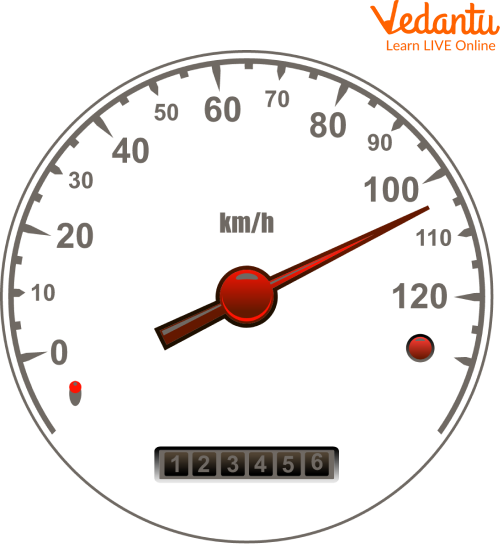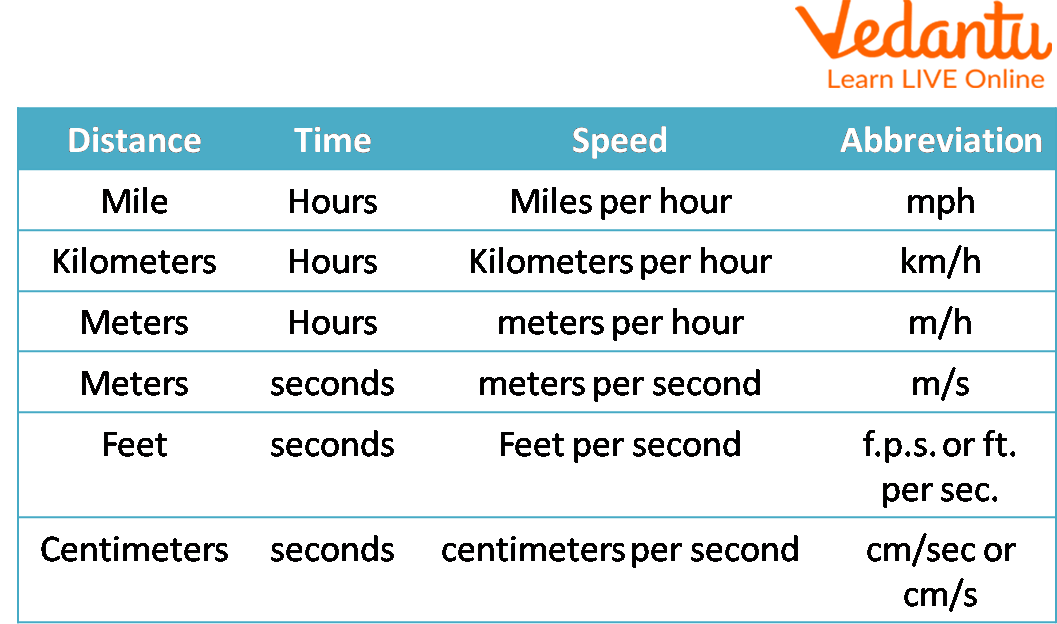Courses
Courses for Kids
Free study material
Free LIVE classes
More

# Speed Distance Time Questions for Class 5LIVE
Join Vedantu’s FREE Mastercalss

## An Introduction to Speed, Distance, and Time

Riya used to come home late after school, cycling at a slower speed, while her friend Diya came home early as she was cycling faster than Riya. Speed refers to how fast something is moving or capable of moving.

In mathematics, speed is measured as the distance travelled/travelled per unit of time. The distance travelled is in kilometres, and the time is in hours, so the unit of measurement for speed is "km" per "hour" or km/h.Speed in Speedometer

## Table of Units in Common Use for Speed

Here, we will see the different terms regarding speed, distance, and time. Also, the abbreviation has been added to make it more readable.Units used for Speed and their Abbreviations

## Solved Example Problems on Speed Distance and Time

Let’s see the speed, distance, and time formula and some solved questions based on it.

### Type 1: Formula for Speed = $\dfrac{\text{Distance (in unit of length)}}{\text{Time (in unit of time)}}$

Example 1: A car covers a distance of $224 \mathrm{~km}$ between Goa and Mumbai in 4 hours. Find its average speed.

Ans: Average Speed $=$ $\dfrac{\text{Total distance covered}}{\text{Total time taken }}$

$\dfrac{224\,Km}{4\,hr}=56\,Km/hr$

Therefore, its average speed is 56 km/hr.

Example 2: The train's speed is $108 \mathrm{~km} / \mathrm{h}$. Find its speed in metres per second.

Ans:

$1 \mathrm{~km}=1000m$

$\text { Speed }=108\left(\frac{\mathrm{Km}}{\mathrm{Hr}}\right)$

Also, we know that 1 minute $=60$ seconds

Therefore, that's 1 hour. $=60 \times 60=3600$ seconds

By using the above stats, we get

$=108\left(\dfrac{1000 \mathrm{~m}}{3600 \mathrm{~s}}\right)$

$=30\left(\dfrac{\mathrm{m}}{\mathrm{s}}\right)$

Therefore, its speed is $=30\left(\dfrac{\mathrm{m}}{\mathrm{s}}\right)$.

Example 3: Latvija covers the first 120 km in 2 hours and the next 180 km in 4 hours. What is his average speed over the entire journey in km/h?

Ans: Total distance travelled = 120 + 180 = 300 km.

Total time taken = 2 + 4 = 6 hours.

Average speed = $\dfrac{\text{Total distance travelled}}{\text{Total time spent}}$

$=\dfrac{300}{6}=50 \mathrm{~km} / \mathrm{h}$

### Type 2: Speed ​​and Distance Formula

Average speed = Total distance travelled / Total time spent

Solved Examples based on Distance and Time:

Example 1: Using the average speed formula, find the average speed of Sam, who covers the first 200 kilometres in 4 hours and the next 160 kilometres in another 4 hours.

Ans: To find the average speed, we need the total distance and the total time.

Total distance covered by Sam = 200 Km + 160 km = 360 km

Total time taken by Sam = 4 hour + 4 hour = 8 hour

Average Speed = $\dfrac{\text{Total distance covered}} {\text{Total time taken}}$

Average Speed = $\dfrac{360}{8}$

= 45 km/hr

Therefore, their average speed of Sam is 45lm/hr.

Example 2: A cyclist rides at $10 \mathrm{~km} / \mathrm{h}$ for 2 hours then at $13 \mathrm{~km} / \mathrm{h}$ for 1 hour. Find its average speed.

Ans:

Distance travelled in the first 2 hours $=10 \times 2=20 \mathrm{~km}$

Distance travelled in the next hour $=13 \times 1=13 \mathrm{~km}$

Total distance travelled $=20+13=33 \mathrm{~km}$

Total time spent $=2+1=3 h r s$

Average speed $=$ $\dfrac{\text{Total distance travelled}}{\text{Total time spent }}$

$=\dfrac{33}{3}=11 \mathrm{~km} / \mathrm{hr}$

### Type 3. Average Speed - If the Travel Time is the Same.

Speed Distance Time problems: In this type of problem, the travel time is always the same.

Example 1: A motorist drives for one hour at an average speed of 45 km/h, and for the next hour at an average speed of 65 km/h. What is your average speed?

Ans: $\dfrac{(45 + 65)}{2}=55 \mathrm{~km} / \mathrm{hr}$

The total distance covered by the car driver in these two hours was $65+45=110 \mathrm{~km}$, and it took two hours.

Therefore your average speed $=55 \mathrm{~km} / \mathrm{h}$.

Example 2: Radha can type 960 letters in 20 mins.

Calculate her typing speed in the:

(a) Words per min.

(b) Words per hour.

Ans:

Words per min. $=\dfrac{960}{20}$

$=48$ words per minute

Words per hour. $=48 \times 60$

$=2880$ words per hour.

### Type 4. Average speed: If the distance covered is the same:

Speed Distance Problems: In this type of problem, the distance covered is always the same.

Example: On the way from the hospital to the Pimpri department, I drive at 30 km/h and back at 45 km/h. What is my average travel speed?

Ans: 37.5 km/h is inappropriate as the time travel is different in both cases, and only the distances are the same. Let the distance between the hospital and the Pimpri ward be x km.

The time spent on the outward journey $=\dfrac{x}{30}$ hour and the time spent on the return journey $=\dfrac{x}{45}$

$\therefore$ Total round trip time $=\dfrac{x}{30}+\dfrac{x}{45}$ $=\dfrac{5 x}{90}$ hours.

Total distance travelled in both directions $=2 \times x \mathrm{km}$

$\therefore$ Average speed $\dfrac{2x}{\dfrac{5x}{90}}=36 \mathrm{kmph}$

## Practice Questions

Let’s see some of the speed distance time questions for Class 5:

Q 1 An aeroplane covers a certain distance in 5 hours at a speed of 240 km/h. To travel the same distance in 1 hour, at what speed must he travel:

Ans: 720 km/hr.

Q 2. How many minutes for the bus per hour? Excluding stops, the speed of a bus is 54 km/h, and including stops, it is 45 km/h.

Ans: 10 min.

Q 3. Determine the time it takes when the distance is 7150 km, and the speed is 780 km/h.

Ans: 9.16 hr

## Summary

Speed tells us how fast something or someone is travelling. You can find the average speed of an object if you know the distance travelled and the time it took. The formula for speed is speed = distance ÷ time. To work out what the units are for speed, you need to know the units for distance and time. In mathematics, speed is measured as the distance travelled per unit of time.

The distance travelled is in kilometres, and the time is in hours, so the unit of measurement for speed is "km" per "hour" or km/h. If the distance is in metres and the time is in minutes, then the speed is 'm' for 'minute' or m/min. You can also read this offline. Just download the speed distance time questions for Class 5 PDF from our website.

Last updated date: 23rd Sep 2023
Total views: 88.5k
Views today: 1.88k

## FAQs on Speed Distance Time Questions for Class 5

Q1. How do we use speed in everyday life?

Ans: The vehicle's speed. How quickly the train is moving. The river is moving at a fluctuating speed. The rate at which water leaves a tap.

Q2. Why are speed, distance, and time important?

Ans: Speed, distance and time refer to the three important variables in physics and maths. Furthermore, these three variables certainly facilitate the solving of several types of problems in Maths.

Q3. Who discovered the formula for speed, namely distance/time?

Ans: Italian physicist Galileo Galilei is credited with being the first to measure speed by taking into account the distance travelled and the time it takes. Galileo defined speed as the distance travelled per unit of time.